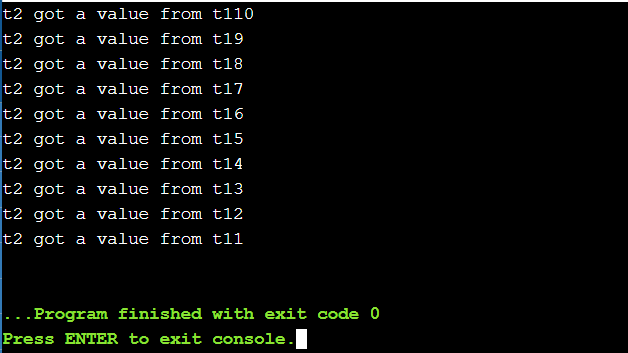# How to wake up a std::thread while it is sleeping?

• Difficulty Level : Hard
• Last Updated : 31 Mar, 2021

In this article, we will discuss how to wake up a std::thread while it is sleeping. It is known that a thread can’t be exited when it is sleeping. So it is woken up using a command as:

std::condition_variable

Below is the pseudo-code to implement the same:

## C++

 `// Custom Class ` `struct` `MyClass { ` ` `  `    ``// Constructor ` `    ``MyClass() ` `        ``: my_thread([``this``]() { ` `            ``this``->``thread``(); ` `        ``}) ` `    ``{ ` `    ``} ` ` `  `    ``// Destructor ` `    ``~MyClass() ` `    ``{ ` `        ``{ ` `            ``std::lock_guard l(m_); ` `            ``stop_ = ``true``; ` `        ``} ` `        ``c_.notify_one(); ` `        ``my_thread.join(); ` `    ``} ` ` `  `    ``// Function that implements the ` `    ``// thread ` `    ``void` `thread``() ` `    ``{ ` `        ``while` `(``this``->wait_for(std::chrono::minutes(2))) ` `            ``SendStatusInfo(some_info); ` `    ``} ` ` `  `    ``// Function to returns false when ` `    ``// the thread is stopped ` `    ``template` `<``class` `Duration> ` `    ``bool` `wait_for(Duration duration) ` `    ``{ ` `        ``std::unique_lock l(m_); ` `        ``return` `!c_.wait_for(l, duration, [``this``]() { ` `            ``return` `stop_; ` `        ``}); ` `    ``} ` ` `  `    ``// Conditions Variable ` `    ``std::condition_variable c_; ` `    ``std::mutex m_; ` `    ``bool` `stop_ = ``false``; ` `    ``std::``thread` `my_thread; ` `}; `

Below is another example to illustrate the same:

std::promise/std::future

The above command can be used as a simpler alternative to the former method. In this case, a Future is not susceptible to false wakes and doesn’t require a mutex for synchronization. Below is the pseudo-code to implement the same:

## C++

 `// Promise Condition ` `std::promise<``void``> pr; ` ` `  `// Start the thread ` `std::``thread` `thr{ ` `        ``[fut = pr.get_future()]{ ` ` `  `            ``// Iterate until the condition ` `            ``// break ` `            ``while` `(``true``){ ` `                ``if` `(fut.wait_for(std::chrono::minutes(2)) ` `                    ``!= std::future_status::timeout) ``return``; ` `} ` `} ` `} ` `; ` ` `  `// When ready to stop ` `pr.set_value(); ` ` `  `// Join the thread ` `thr.join(); `

Below is the program to illustrate the above concepts:

## C++

 `// C++ program to illustrate the waking ` `// of the thread while it is sleeping ` `#include ` `using` `namespace` `std; ` ` `  `std::deque<``int``> q; ` `std::mutex mu; ` `std::condition_variable cond; ` ` `  `// Function to create the thread 1 ` `void` `function_1() ` `{ ` `    ``// Initialize a counter variable ` `    ``int` `count = 10; ` ` `  `    ``// Iterate until count is positive ` `    ``while` `(count > 0) { ` ` `  `        ``// Mutex ` `        ``std::unique_lock locker(mu); ` ` `  `        ``// Push the current count in ` `        ``// the dequeue ` `        ``q.push_front(count); ` `        ``locker.unlock(); ` `        ``cond.notify_one(); ` ` `  `        ``// If there is any waiting thread ` `        ``// then notify that thread ` `        ``std::this_thread::sleep_for( ` `            ``chrono::seconds(1)); ` ` `  `        ``// Decrement the count ` `        ``count--; ` `    ``} ` `} ` ` `  `// Function to create the thread 2 ` `void` `function_2() ` `{ ` `    ``// Initialize a variable to get ` `    ``// the data from the deque ` `    ``int` `data = 0; ` ` `  `    ``while` `(data != 1) { ` ` `  `        ``std::unique_lock locker(mu); ` ` `  `        ``cond.wait(locker, []() { ` `            ``return` `!q.empty(); ` `        ``}); ` ` `  `        ``// False Waking of thread ` `        ``data = q.back(); ` `        ``q.pop_back(); ` `        ``locker.unlock(); ` ` `  `        ``// Print the message ` `        ``cout << ``"t2 got a value from"` `                ``" t1"` `             ``<< data << ``'\n'``; ` `    ``} ` `} ` ` `  `// Driver Code ` `int` `main() ` `{ ` `    ``// Create thread 1 ` `    ``std::``thread` `t1(function_1); ` ` `  `    ``// Create thread 2 ` `    ``std::``thread` `t2(function_2); ` ` `  `    ``// Join the threads ` `    ``t1.join(); ` `    ``t2.join(); ` ` `  `    ``return` `0; ` `} `

Output:My Personal Notes arrow_drop_up
Recommended Articles
Page :